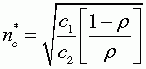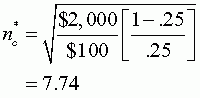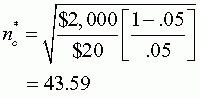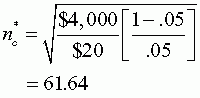# Exercises and Discussion Questions

6.1       Given the information in Case Study 6.2, what would be the optimum cluster size if ρ is .25 instead of .05? What would be the optimum cluster size if ρ is .05 but the variable cost per observation (c2) is $20 instead of$100? What would be the optimum cluster size if ρ is .05, c2 is $20, and the fixed cost per cluster (c1) is$4,000 instead of $2,000? We use Equation 6.2 to solve for optimum cluster size. That equation is:Given the information in this exercise, if ρ is .25 instead of .05, the optimum cluster size would be:If ρ is .05 but the variable cost per observation is$20 instead of $100, the optimum cluster size would be:If ρ is .05, the variable cost per observation is$20, and the fixed cost per cluster is $4,000 instead of$2,000, the optimum cluster size would be:6.2       Assume that you want to draw a statewide multistage cluster sample of residents in your state, and the first stage is to select 10 counties (or 10 places if your state does not have 10 counties). Draw a PPS sample of 10 counties as we did in Section 6.5.2.

There is no set answer to this question. Just follow the procedures given in Section 6.5.2.